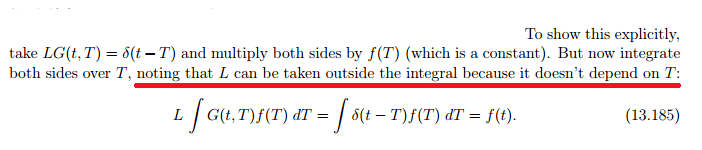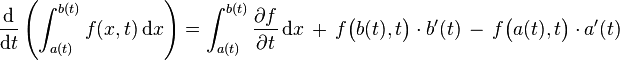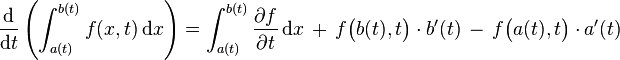# Differentiation and integration

## Homework Statement

Hi,I saw a statement in my physics notes like this(Anyway it is a maths problem):where L is a general differential operator.G is a green's function(I guess it is irrelevant)
My question is related to the red line:
Suppose we have this:
∂/∂x ∫ f(x-y)g(y) ∂y
is it generally true that
∂/∂x ∫ f(x-y)g(y) ∂y = ∫ ∂/∂x [ f(x-y)g(y) ] ∂y ?

## Homework Equations

Please answer it as simply as you can...Since I have not done multivariable calculus...(Though I would be happy to check it out if there is a theorem related to this.)

## The Attempt at a Solution

If the above is simplified to ∂/∂x ∫ f(x)g(y) ∂y ,then ∂/∂x [f(x) ∫ g(y) ∂y]
⇒ ∫ ∂/∂x [ f(x) ] g(y) ∂y
⇒ ∫ ∂/∂x [ f(x)g(y) ] ∂y

But I dont know what to do with the (x-y) term..

Thanks

ShayanJ
Gold Member
The general formula is:But you don't need this. You only need to use the properties of Dirac delta, i.e. ## \int \delta(x-y) f(y) dy=f(x)##.

The general formula is:But you don't need this. You only need to use the properties of Dirac delta, i.e. ## \int \delta(x-y) f(y) dy=f(x)##.
Thanks :) Is there a name for this formula?

ShayanJ
Gold Member
•davon806